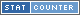Www.WorldHistory.BizAdd New Article
Www.WorldHistory.Biz
SundriesContact
 Login *: Password *:Register7-10-2015, 08:04

# Rooms of Visit

The geometry of the room of visit in the first derivation is presented in Fig. 8.17.

Flow rates Q between 2 and 25 kg/s are studied. A 3D mesh of approximately 170 103 hexahedral cells is generated.Fig. 8.17 Geometry of the room of visit in the first derivation, drawn with GambitFig. 8.18 Velocity vector field in a horizontal plane 4 cm above the bottom of the room of visit in the first derivation. Q = 10 kg/s

The level of water in the room of visit is roughly determined from the measurement of the height of a calcareous deposit (+/- 30 cm). An accurate determination of this level is not essential, as the energy loss in the room of visit is not very sensitive to it.

The standard k-e turbulence model is first used. Once the convergence is reached, the turbulence model is switched to a k-e RNG model. Further iterations are performed, until convergence is reached again.

In Fig. 8.18, the simulated velocity vector field in a horizontal plane in the room of visit (4 cm from the bottom) is presented, for Q = 10 kg/s. The presence of a large stagnant zone can be observed.

When a simulation has been realized, the volumetric energy difference between cross-sections A and B, defined in Fig. 8.18, is reported. In Fig. 8.19, EA - EB is plotted as a function of Q2. A linear relationship between the energy loss and Q2 is observed. A linear regression of these data gives CRi = 6.9 (using Equation (8.9) and the fact that Q A is measured equal to 190 cm2).Fig. 8.19 Energy loss in rooms of visit, as functions of Q2. Energy loss in the decanter in the second derivation, as a function of Q2

8.3.1.2 Derivation 2

Flow rates Q between 2 and 30 kg/s are studied. The flow in the room of visit is turbulent. A 3D mesh of approximately 170x103 hexahedral cells is generated. A k-e standard model of turbulence is used here.

In Fig. 8.20, the structure of the flow in a horizontal plane in the room of visit (12 cm from the bottom) is presented, for Q = 8 kg/s. The presence of a large vortex can be observed.Fig. 8.20 Velocity vector field in a horizontal plane 12 cm above the bottom of the room of visit in the second derivation. Q = 8 kg/s

When a simulation has been realized, the volumetric energy difference between cross-sections A and B, defined in Fig. 8.20, is reported. In Fig. 8.19, this energy loss is plotted as a function of Q2. A linear relationship between this energy loss and Q2 can be observed. The energy drop coefficient of the flow in the room of visit is written CR2. CR2 = 1.1 can be extracted from the data of Fig. 8.19 (using the fact that the area of the section of the connection between the room of visit and the aqueduct is equal to 48 cm2).

It can be observed that, at a same Q, the energy loss in the room of visit in the second derivation is more than twice the energy loss in the room of visit in the first one (Fig. 8.19). A part of this significant difference is due to the presence of the large vortex in the room of visit in the second derivation.World History